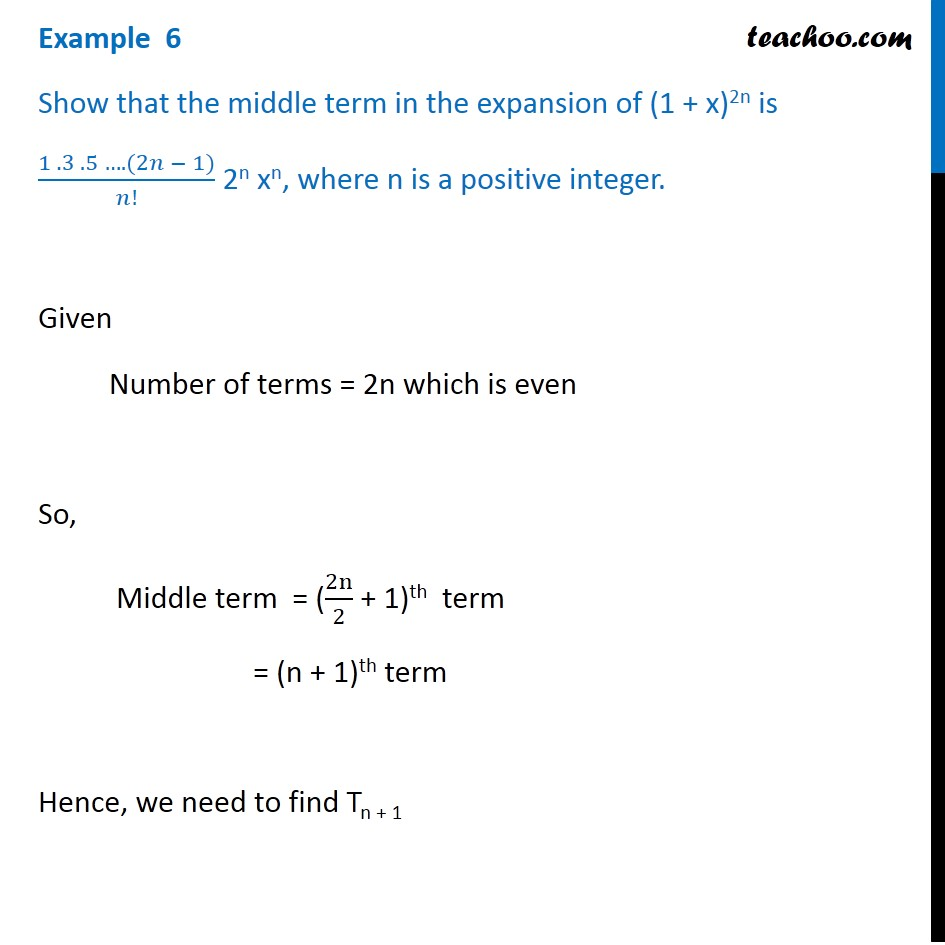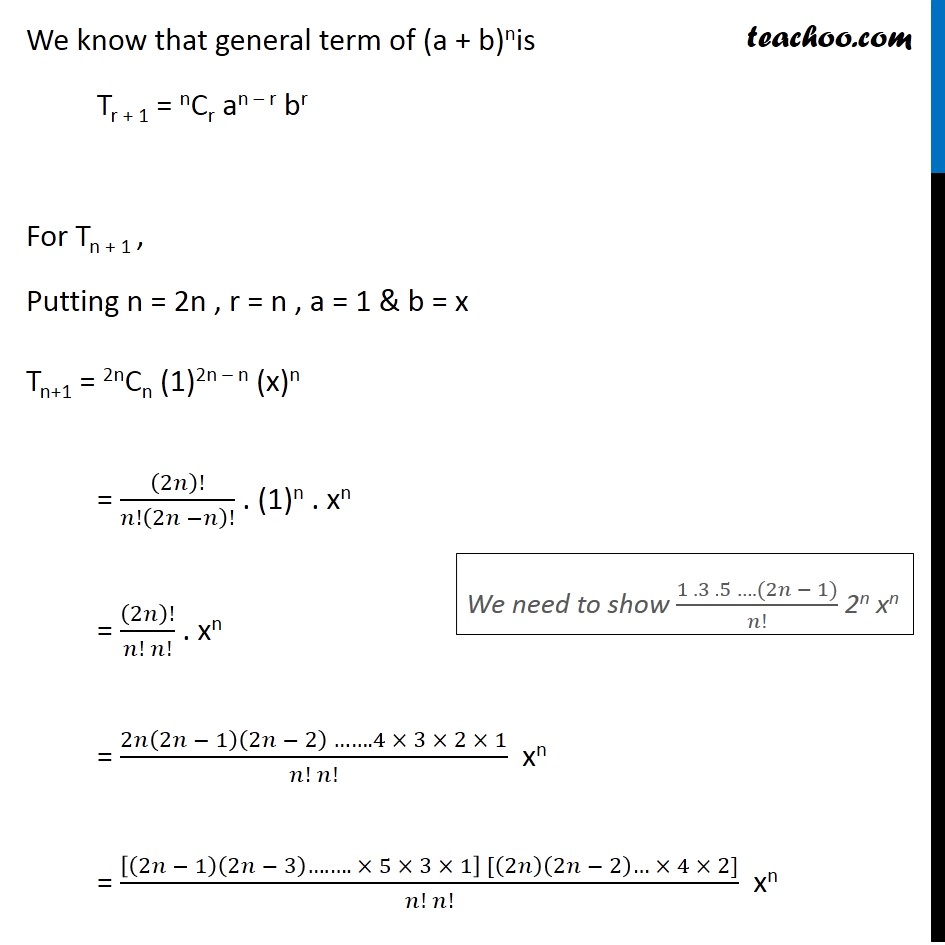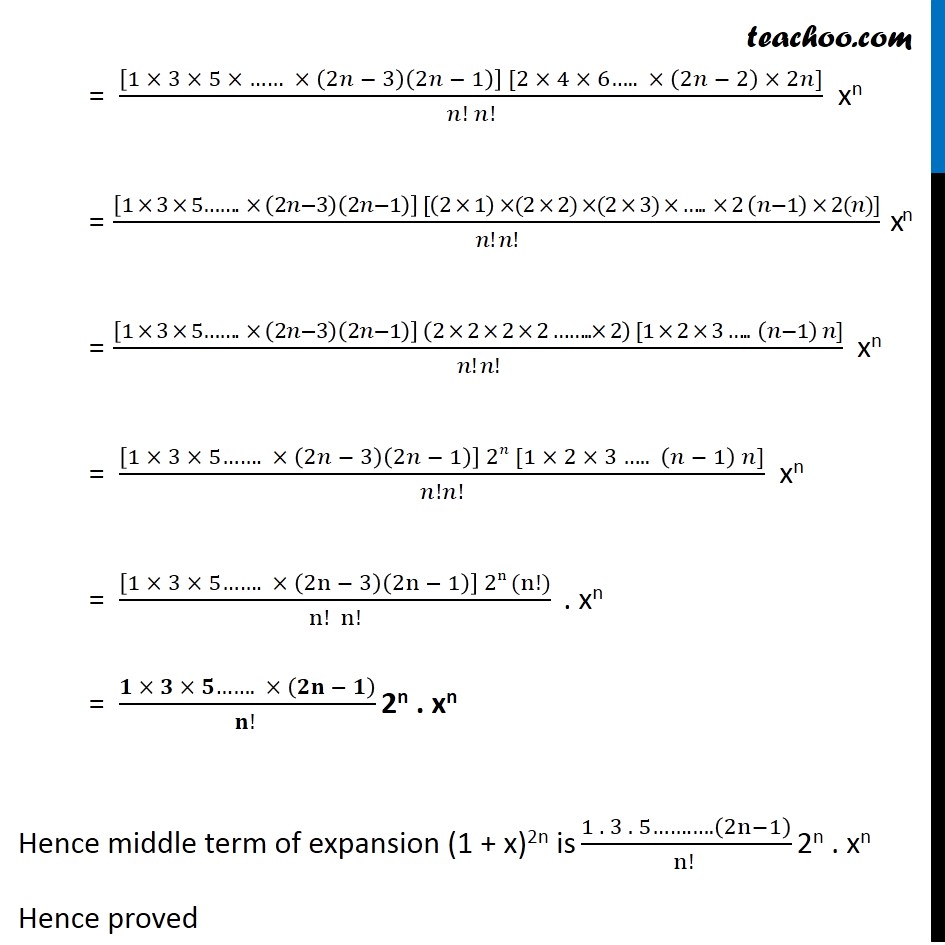Examples

Chapter 7 Class 11 Binomial Theorem
Serial order wiseLearn in your speed, with individual attention - Teachoo Maths 1-on-1 Class

### Transcript

Question 2 Show that the middle term in the expansion of (1 + x)2n is (1 . 3 . 5 …. (2𝑛 − 1))/𝑛! 2n xn, where n is a positive integer. Given Number of terms = 2n which is even So, Middle term = (2n/2 + 1)th term = (n + 1)th term Hence, we need to find Tn + 1 We know that general term of (a + b)nis Tr + 1 = nCr an – r br For Tn + 1 , Putting n = 2n , r = n , a = 1 & b = x Tn+1 = 2nCn (1)2n – n (x)n = (2𝑛)!/𝑛!(2𝑛 −𝑛)! . (1)n . xn = (2𝑛)!/(𝑛! 𝑛!) . xn = (2𝑛(2𝑛 − 1)(2𝑛 − 2) ……. 4 × 3 × 2 × 1)/(𝑛! 𝑛!) xn = ([(2𝑛 − 1)(2𝑛 − 3)….…. × 5 × 3 × 1] [(2𝑛)(2𝑛 − 2)… × 4 × 2])/(𝑛! 𝑛!) xn We need to show (1 . 3 . 5 …. (2𝑛 − 1))/𝑛! 2n xn = ([1 × 3 × 5 × …… × (2𝑛 − 3)(2𝑛 − 1)] [2 × 4 × 6….. × (2𝑛 − 2) × 2𝑛])/(𝑛! 𝑛!) xn = ([1 × 3 × 5……. × (2𝑛−3)(2𝑛−1)] [(2 × 1) ×(2 × 2) ×(2 × 3) × ….. × 2 (𝑛−1) × 2(𝑛)])/(𝑛! 𝑛!) xn = ([1 × 3 × 5……. × (2𝑛−3)(2𝑛−1)] (2 × 2 × 2 × 2 ……..× 2) [1 × 2 × 3 ….. (𝑛−1) 𝑛])/(𝑛! 𝑛!) xn = ([1 × 3 × 5……. × (2𝑛 − 3)(2𝑛 − 1)] 2𝑛 [1 × 2 × 3 ….. (𝑛 − 1) 𝑛])/𝑛!𝑛! xn = ([1 × 3 × 5……. × (2n − 3)(2n − 1)] 2n (n!))/(n! n!) . xn = (𝟏 × 𝟑 × 𝟓……. × (𝟐𝐧 − 𝟏))/(𝐧! ) 2n . xn Hence middle term of expansion (1 + x)2n is (1 . 3 . 5……. ….(2n−1))/(n! ) 2n . xn Hence proved